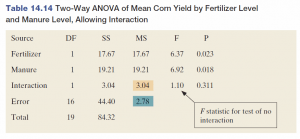20
Nov 16

## The pearls of AP Statistics 36ANOVA: the artefact that survives because of the College Board

### Why ANOVA should be dropped from AP Statistics

1. The common argument in favor of using ANOVA is that "The methods introduced in this chapter [Comparing Groups: Analysis of Variance Methods] apply when a quantitative response variable has a categorical explanatory variable" (Agresti and Franklin, p. 680). However, categorical explanatory variables can be replaced by indicator (dummy) variables, and then regression methods can be used to study dependences involving categorical variables. On p. 695, the authors admit that "ANOVA can be presented as a special case of multiple regression".
2. In terms of knowledge of basic statistical ideas (hypothesis testing, F statistics, significance level), ANOVA doesn't add any value. Those, who have mastered these basic ideas, will not have problems learning ANOVA at their workplace if they have to. There is no need to burden everybody with this stuff "just in case".
3. The explanation of ANOVA is accompanied with definitions of the within-groups variance estimate and between-groups variance estimate (Agresti and Franklin, p. 686). Even in my courses, where I give a lot of algebra, the students don't get them unless they do a couple of theoretical exercises. At the AP Stats level, the usefulness of these definitions is nil.
4. The requirement to remember how the F statistics and degrees of freedom are calculated, for the purpose of being able to interpret just one table with output from a statistical package, doesn't make sense. In my book, I have a whole chapter on ANOVA, with most derivations, and I don't remember a thing. Why torture the students?
5. In the 90 years since R. Fisher has invented ANOVA, many other, more precise and versatile, statistical methods have been developed.

### Conclusion

There are two suggestions

1) Explain just the intuition and then jump to the interpretation of output, indicating the statistic to look at, as in Table 14.14.2) The theory of ANOVA is useful for two reasons: there is a lot of manipulation with summation signs and there is a link to regressions. Learning all this may be the only justification to study ANOVA with definitions. In my classes, this takes 6 hours.